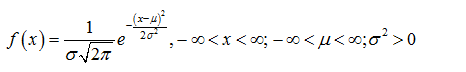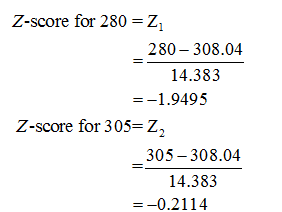# The drive distance (in yards) for professional golfers is normally distributed with a mean of 308.04 yards and a standard deviation of 14.383 yards. What percentage of golfers has a drive distance between 280 and 305 yards? z1 = z2 = percentage=

Question
1 views

The drive distance (in yards) for professional golfers is normally distributed with a mean of 308.04 yards and a standard deviation of 14.383 yards. What percentage of golfers has a drive distance between 280 and 305 yards?

z1 =

z2 =

percentage=

check_circle

Step 1

The normal distribution:

A continuous random variable X is said to follow normal distribution with mean µ and standard deviation σ if the probability density function of X is,Step 2

Calculating the indicated distance:

The drive distance for professional golfers is normally distributed with mean 308.04 and standard deviation of 14.383. The required z-scores can be calculated as follows:...

### Want to see the full answer?

See Solution

#### Want to see this answer and more?

Solutions are written by subject experts who are available 24/7. Questions are typically answered within 1 hour.*

See Solution
*Response times may vary by subject and question.
Tagged in

### Statistics